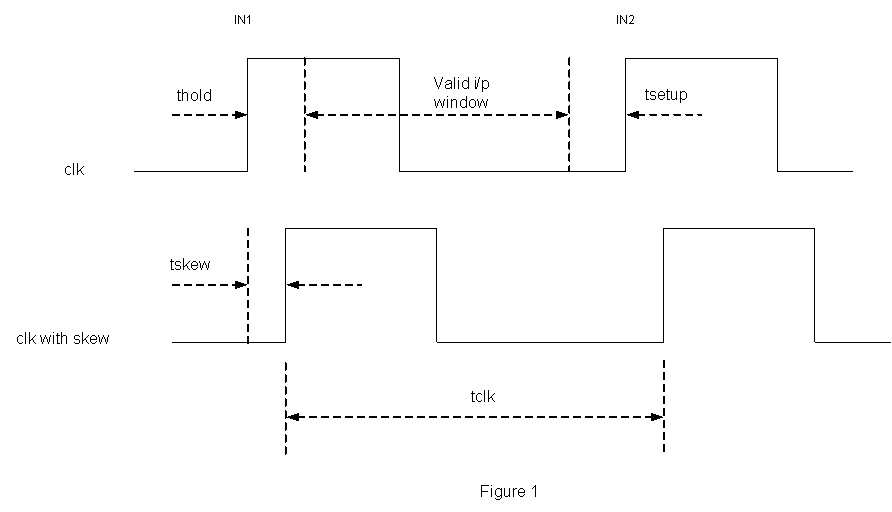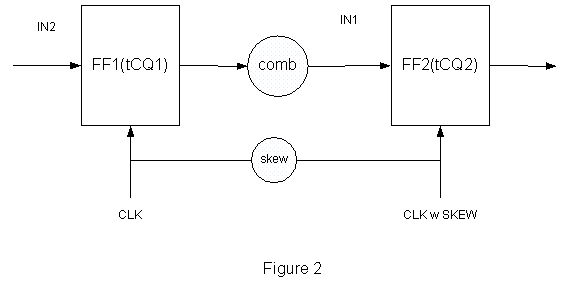## Setup time, Hold time

### Setup time, Hold time .

SETUP TIME & HOLD TIME EQUATIONS

This section derives the equation for valid input window for a flip-flop to avoid set up and hold time violations.Assume tskew = 0, and FF2 is processing IN1, and FF1 is processing IN2,

IN2 should not reach FF2 before thold of FF2 to avoid Meta stability at FF2,

Hold time -> tCQ (FF1) + tcomb > thold (FF2)--- Eq.1

At the same time, IN2 should reach FF2 before setup time of FF2,

Setup time -> tCQ (FF1) + tcomb < tclk - tsetup (FF2)--- Eq.2

If tskew != 0, equations will be modified in the following way,

Hold time -> tCQ (FF1) + tcomb > thold (FF2) + tskew --- Eq.3

Setup time -> tCQ (FF1) + tcomb < tclk + tskew - tsetup (FF2) --- Eq.4

In Eq.3, skew is added to hold time, becomes effective hold time. In Eq.4, skew is added to clock period, becomes effective period or you can think, effective set up time is decreased by skew. This discussion is just for the purpose of our understanding. From equations, positive skew is good for setup time and bad for hold time.

Home